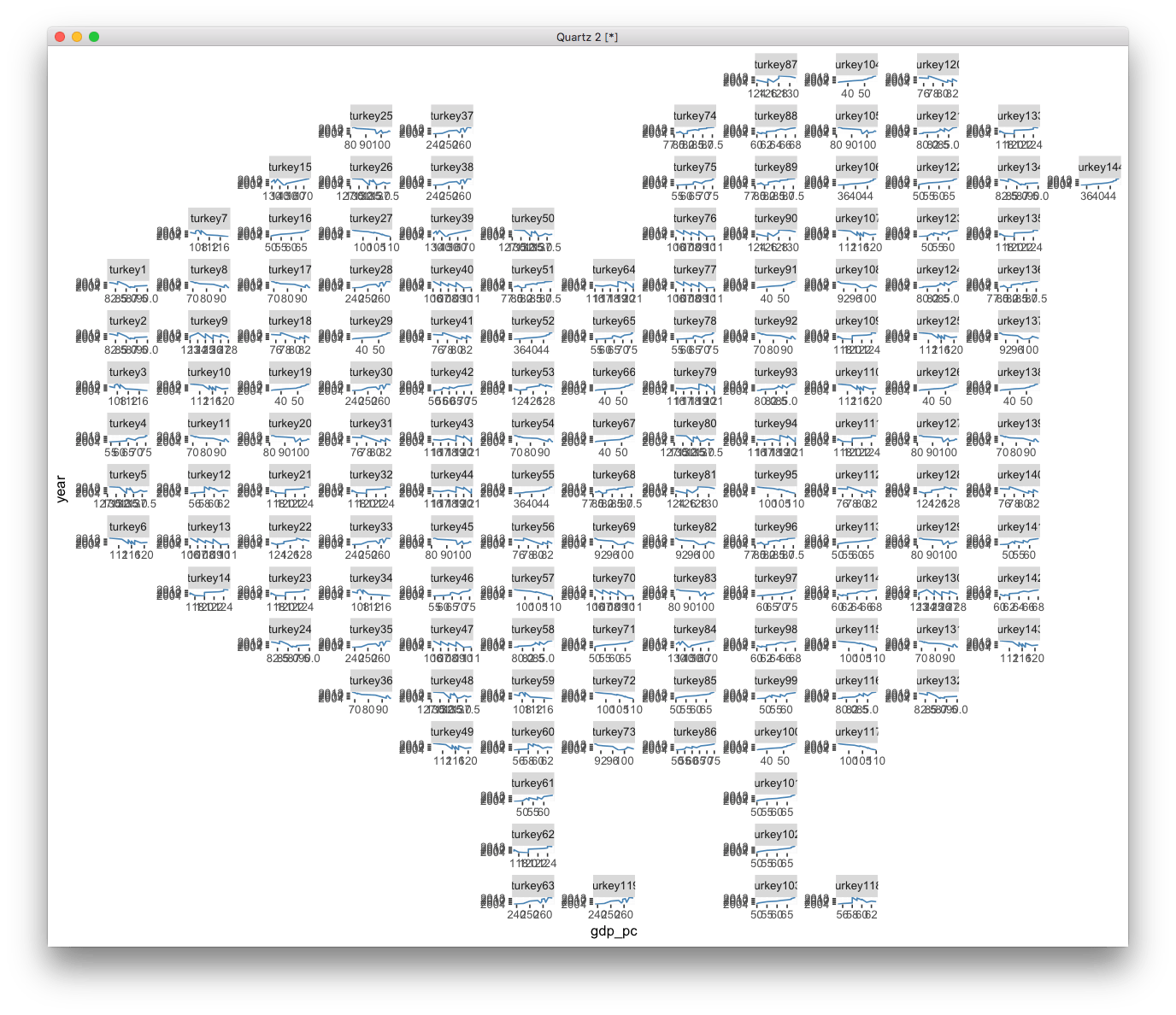Want to share your content on R-bloggers? click here if you have a blog, or here if you don't.

I just discovered an interesting `ggplot2` extension, `geofacet`, that supports arranging facet panels that mimics geographic topoloty.

After playing with it, I realized that it is not only for visualizing `geo`-related data, but also can be fun for presenting data to mimics pixel art.Here is an example using the Turkey shape:

```Turkey <- read.csv("http://pages.iu.edu/~cdesante/turkey.csv")
Turkey = Turkey[, -3]
colnames(Turkey)[2:1] = c("row", "col")
Turkey\$row = max(Turkey\$row) - Turkey\$row +1
Turkey\$name <- Turkey\$code <- paste0('turkey', 1:nrow(Turkey))

require(ggplot2)
require(geofacet)
x <- split(eu_gdp, eu_gdp\$code)
x <- x[sample.int(length(x), nrow(Turkey), replace=T)]
for (i in 1:length(x)) {
x[[i]]\$code = Turkey\$code[i]
}
y <-  do.call(rbind, x)

p1 <- ggplot(y, aes(gdp_pc, year))+ geom_line(color="steelblue") +
facet_geo(~code, grid=Turkey, scales='free')
print(p1)
``````p1 + theme_void() + theme(strip.text.x = element_blank())
```